# How to Count or Sum Cells With Certain Color in Excel

This tutorial will demonstrate you how to count or sum cells of a certain background color using VBA.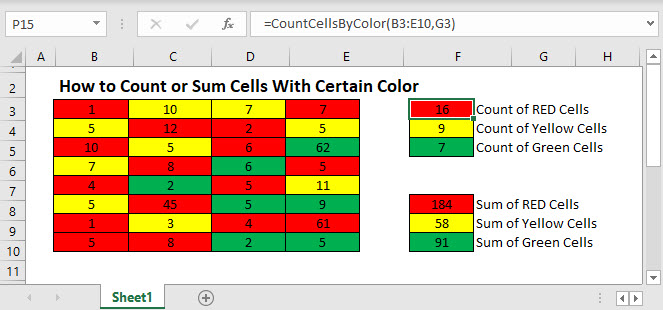Consider the following range in Excel.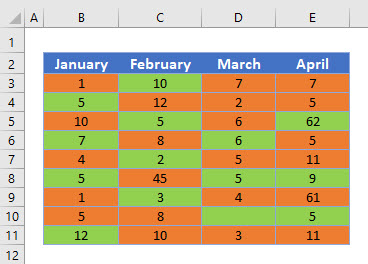There is no built in Excel function to count colored cells. Instead we must create a User-Defined Function using VBA.

## Count Cells – Create a VBA Custom Function

Counting how many cells are of a certain color using VBA is a matter of creating a user-defined function (UDF) that will loop through all the cells in the range, and determine if the background color of each cell matches the background color that you are testing for, and then using that function in the Excel worksheet.

In VBA, we create a function to count selected cells.

``````Function CountCellsByColor(rng As Range, ColorCell As Range) As Double
Dim dblCount As Double
Dim rngCell As Range
'Loop throught each cell in the range
For Each rngCell In rng
'check to see if the interior color is the same color as the cell you have selected
If rngCell.Interior.Color = ColorCell.Interior.Color Then
If IsNumeric(rngCell.Value) = True Then
'increase the count by 1 if the color is correct.
dblCount = dblCount + 1
End If
End If
Next
'Return the value to Excel
CountCellsByColor = dblCount
End Function
``````

Then use this function in the worksheet to return the value.

``=CountCellsByColor(B3:E11,G5)``
1. Click in the orange cell in G4, and click Insert Function.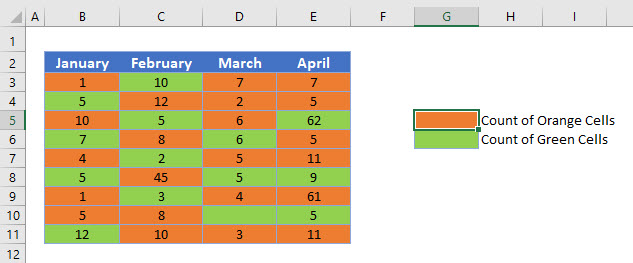1. Select User Defined as the category, and then select CountCellsByColor as the function to use.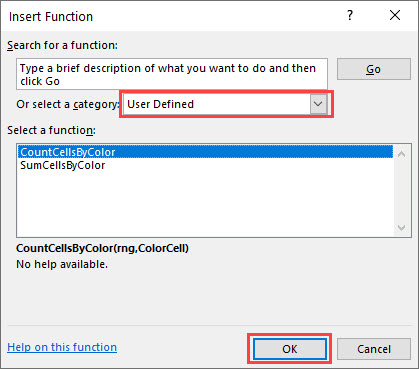1. Click OK.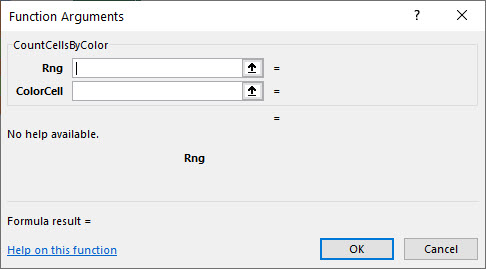1. Highlight the range that contains all the colored cells.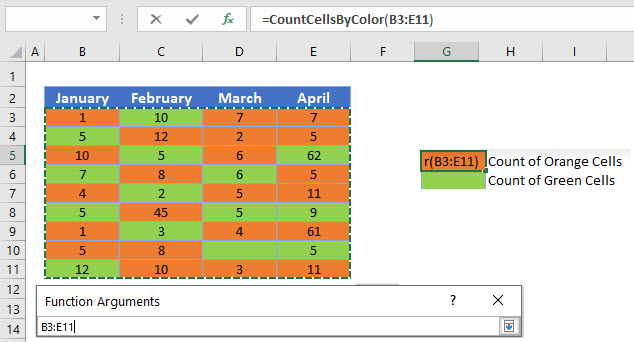1. Select the ColorCell, and then Click OK.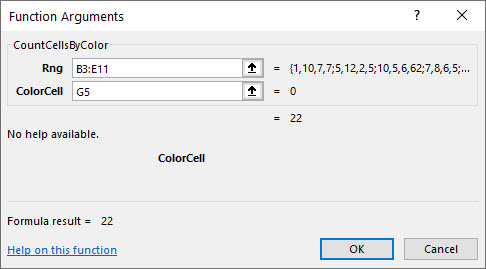Repeat the process to Count the cells with a Green Background color.

``=CountCellsByColor(B3:E11,G6)``

## Sum Cells – Create a Custom VBA Function

We create a similar custom function in VBA to sum the values of the cells of a certain color.

``````Function SumCellsByColor(rng As Range, ColorCell As Range) As Double
Dim dblSum As Double
Dim rngCell As Range
'Loop throught each cell in the range
For Each rngCell In rng
'check to see if the interior color is the same color as the cell you have selected
If rngCell.Interior.Color = ColorCell.Interior.Color Then
If IsNumeric(rngCell.Value) = True Then
dblSum= dblSum + rngCell.Value
End If
End If
Next
'Return the value to Excel
SumCellsByColor = dblSum
End Function
``````

We would then once again use this function in the Worksheet to sum up the required cells.

``=SumCellsByColor(B3:E11,G8)``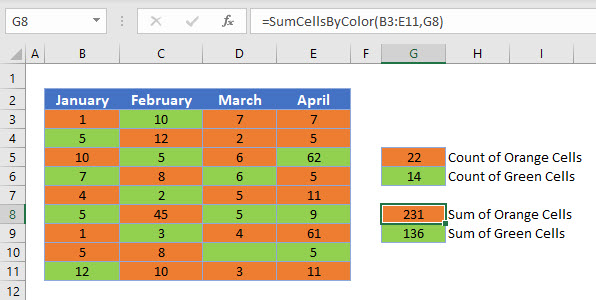### Excel Practice Worksheet

Practice Excel functions and formulas with our 100% free practice worksheets!